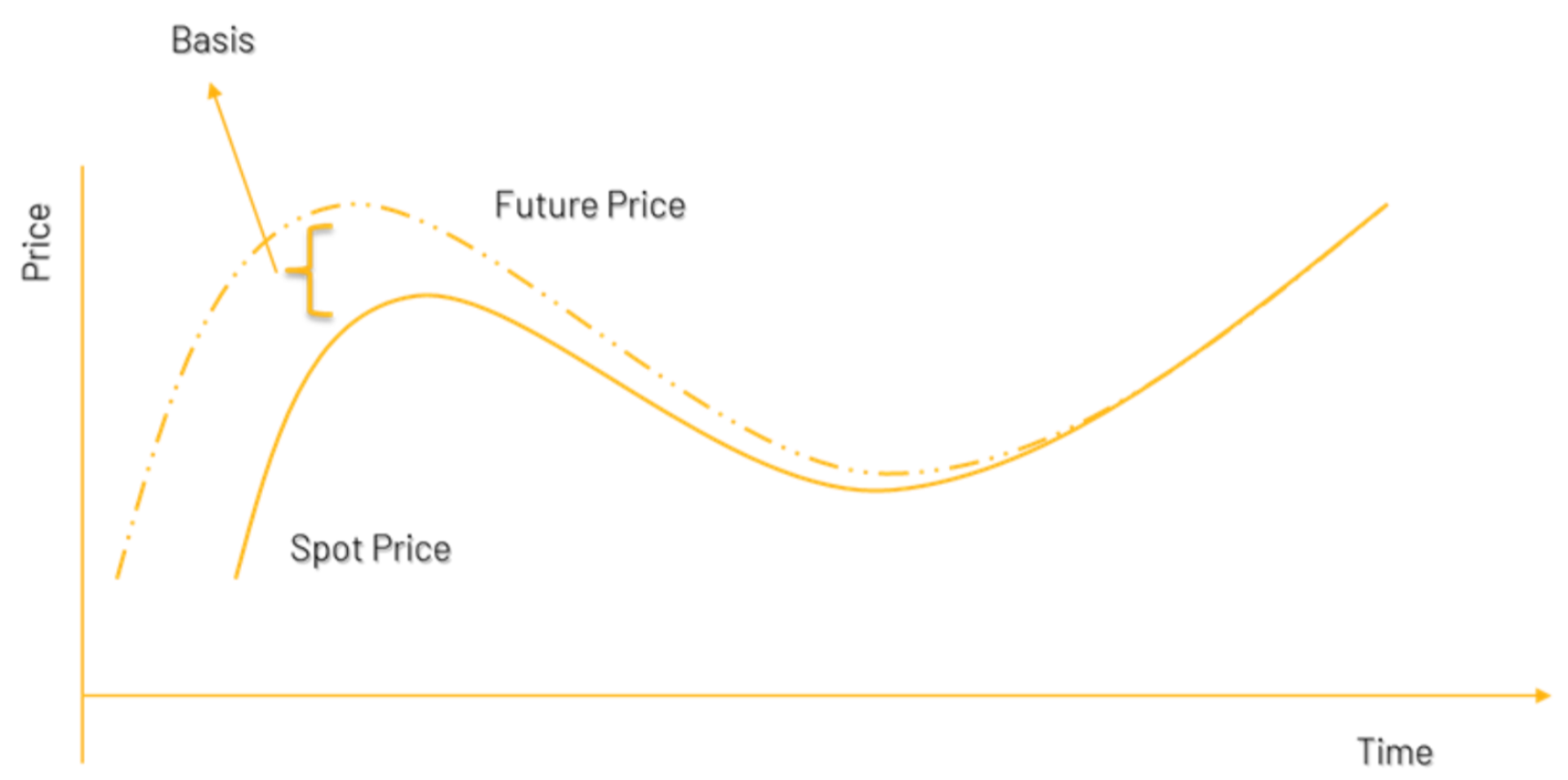Nifty 50 Futures - Nifty futures live Analysis with Quantsapp | QuantsappFutures Pricing

# Futures PricingFutures Pricing

# Futures Pricing

The characteristics of the underlying asset influence the pricing of futures contract based on that asset. It has been difficult to crystallise on a single model for pricing of futures, because different underlying assets have different demand and supply patterns, different characteristics and cash flow patterns. However, one of the well-published and recognised models for futures pricing is Cash and Carry Model.

Cash and carry model of futures pricing works under certain assumptions. The important assumptions are stated below:

1. Underlying asset is available in abundance in cash market.
2. There is no seasonality in demand and supply of underlying asset.
3. Warehousing/storage of underlying asset is feasible/non-perishable.
4. Underlying asset can be sold short.
5. No transaction costs.
6. No taxes.

[This is not an exhaustive list of the assumptions of the model]

When an underlying asset is not storable/ perishable i.e. the asset is not easy to warehouse/hold, then one cannot carry the asset to the future. The cash and carry model is not applicable to these types of perishable underlying assets.

## Cost of Carry

Cost of Carry model defines the relationship between futures prices and spot prices. It measures the warehousing/storage cost (in commodity markets) plus the interest cost associated with carrying the asset till expiration of the futures contract. Of course, other income during the holding period of the futures contract, is subtracted from the cost of carry. Underlying asset like securities (equity or bonds) may have certain inflows, like interest on debt instruments and dividend on equity, during the holding period. For equity derivatives, carrying cost is the interest paid to finance the purchase, reducing dividend earned, as dividend is income and cost of carry is an outgo.

Therefore, the formula for Futures, considering the cost of carry model can be written as:

Ideal Price of Futures = Spot + Cost of Carry – Inflows

F = S*(1+r-q)t

Where F is fair price of the futures contract, S is the Spot price of the underlying asset, q is expected return during holding period t (in years) and r is cost of carry. Note that r and q are annualised figures in percentage. This formula can also be used to evaluate the ideal Nifty future price.

# Basis of a futures contract

The difference between the spot price and the futures price is called basis. If the futures price is greater than spot price, basis for the underlying asset is negative. Similarly, if the spot price is greater than futures price, basis for the underlying asset is positive.

It is also important to understand that the basis difference between one- and two-month futures contract should essentially be equal to the cost of carrying the underlying asset between first and second month. This is fundamentally linked with the cost of carry pricing model.To study the basis behaviour of Nifty, Bank Nifty and other Futures & Option stocks, Quantsapp, India’s option analytics platform can help.

The screen shots ahead show the basis behaviour of Nifty in terms of the actual basis and also basis%.

## FAQs

### When should you buy futures?

When a trader desires to take financial leverage and expects the underlying like Nifty or underlying F&O stock to be strongly bullish, can go ahead and buy futures.

### When should you buy futures?

Ideal Price of Futures = Spot + Cost of Carry – Inflows
F = S*(1+r-q)^t
Where F is fair price of the futures contract, S is the Spot price of the underlying asset, q is expected return during holding period t (in years) and r is cost of carry. Note that r and q are annualised figures in percentage.

### What factors affect futures prices?

Futures prices are affected by the demand and supply of the financial asset which is the underlying. The cost of carry model is used to determine the prices of futures from the underlying spot prices, which uses interest rates (carry cost) and possible cash inflows.